Sie sind hier: ICP » R. Hilfer » Publikationen

# 3 Saturation Overshoot

## 3.1 Experimental Observations

[2328.2.1] Infiltration experiments  on constant flux imbibition into a very dry porous medium report existence of non-monotone travelling wave profiles for the saturation [55, 62]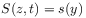(20)

as a function of time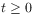and position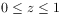along the column. [2328.2.2] Here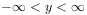denotes the similarity variable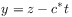(21)

and the parameter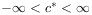is the constant wave velocity. [2328.2.3] From here on all quantitites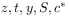are dimensionless. [2328.2.4] The relation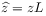(22a)

defines the dimensional depth coordinate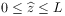increasing along the orientation of gravity. [2328.2.5] The length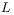is the system size (length of column). [2328.2.6] The dimensional time is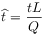(22b)

where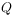denotes the total (i.e. wetting plus nonwetting) spatially constant flux through the column in m/s (see eq.  (13)). [2328.2.7] The dimensional similarity variable reads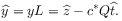(22c)

[2328.2.8] Experimental observations show fluctuating profiles with an overshoot region [59, 73, 54, 55, 74, 75]. [2328.2.9] It can be viewed as a travelling wave profile consisting of an imbibition front followed by a drainage front.

## 3.2 Mathematical Formulation in d=1

[2328.3.1] The problem is to determine the height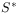of the overshoot region (=tip) and its velocity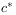given an initial profile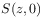, the outlet saturation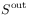, and the saturation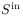at the inlet as data of the problem. [2328.3.2] Constantand constantare assumed for the boundary conditions at the left boundary. [2328.3.3] Note, that this differs from the experiment, where the flux of the wetting phase and the pressure of the nonwetting phase are controlled.

[2328.4.1] The leading (imbibition) front is a solution of the nondimensionalized fractional flow equation (obtained from eq.  (17) for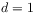)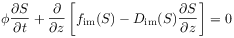(23a)

[page 2329, §0]    while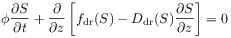(23b)

must be fulfilled at the trailing (drainage) front. [2329.0.1] Here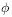is the porosity and the variables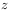and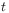have been nondimensionalized using the system sizeand the total flux. The latter is assumed to be constant. [2329.0.2] The functions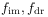are the fractional flow functions for primary imbibition and secondary drainage. [2329.0.3] They are given as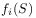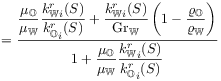(24a)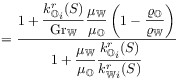(24b)

with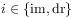and the dimensionless gravity number [76, 37]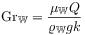(25)

defined in terms of total flux, wetting viscosity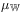, density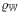, acceleration of gravity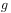and absolute permeability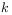of the medium. [2329.0.4] The functions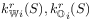withare the relative permeabilities. [2329.0.5] The capillary flux functions for drainage and imbibition are defined as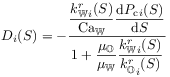(26)

withand a minus sign was introduced to make them positive. [2329.0.6] The functions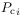are capillary pressure saturation relations for drainage and imbibition. [2329.0.7] The dimensionless number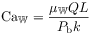(27)

is the macroscopic capillary number [76, 37] with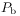representing a typical (mean) capillary pressure at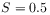(see eq.  (34)). [2329.0.8] For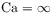one has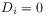and eqs. (23) reduce to two nondimensionalized Buckley-Leverett equations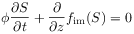(28a)

for the leading (imbibition) front and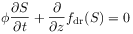(28b)

for the trailing (drainage) front.

[page 2330, §1]

## 3.3 Hysteresis

[2330.1.1] Conventional hysteresis models for the traditional theory require to store the process history for each location inside the sample [77, 78, 79]. [2330.1.2] Usually this means to store the pressure and saturation history (i.e. the reversal points, where the process switches between drainage and imbibition). [2330.1.3] A simple jump-type hysteresis model can be formulated locally in time based on eq.  (23) as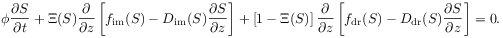(29)

[2330.1.4] Here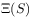denotes the left sided limit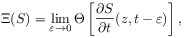(30)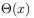is the Heaviside step function (see eq.  (35)), and the parameter functions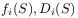with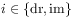require a pair of capillary pressure and two pairs of relative permeability functions as input. [2330.1.5] The relative permeability functions employed for computations are of van Genuchten form [80, 81]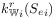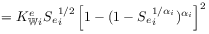(31a)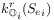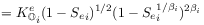(31b)

withand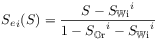(32)

the effective saturation. [2330.1.6] The resulting fractional flow functions with parameters from Table 1 are shown in Figure 1b. [2330.1.7] The capillary pressure functions used in the computations are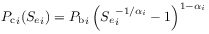(33)

with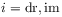and the typical pressurein (27) is defined as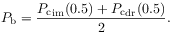(34)

[2330.1.8] Equation (29) with initial and boundary conditions for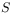and an initial condition for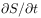is conjectured to be a well defined semigroup of bounded operators on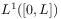on a finite interval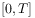of time. [2330.1.9] The conjecture is supported by the fact that each of the equations (23) individually defines such a semigroup, and because multiplication byor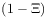are projection operators.